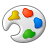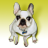# Help: Calculate the shortest FLYING route to fly a list of coordinates

3908
8
01-24-2013 12:06 PMby
New Contributor
Hi all
I'm new to this, and I have a problem I need to solve, and would love to get some help.

I have a spread sheet listing a big number of locations (coordinates). I want to find a simple way to add all locations to a map (that part I know how to do) and then have the software to calculate the shortest FLYING route to fly all to all this locations. It???s like a Traveling Salesman Problem. It need to be straight lines ???as a bird fly???, passing all locations in the best order so total distance is the shortest. I would like it to calculate and present the distance between each leg, and total distance as well.

All route solutions I have found so fare is for finding the best route in a road systems, that don't help me :U
I would really appreciate some help 🙂 And again, I???m new to this so please keep it simple 🙂
Tags (2)
8 RepliesbyEsri Notable Contributor
Use the Points to Line tool to generate lines between the points.  Use a sort field to order the lines as they're created.  You can then create a field and use the Calculate Geometry function to calculate the length of all line features.by
New Contributor
Thanks for that reply. I'm really new to this, so it took  me a while to even get a line beetween the points. But I have mangened to do that now. But the sorting I do not know how to fix.

I want it to calculate the order of point that generate the shortest total distance. The sorting in point to line don't allow for that.
Any help with that part?

ThanksOccasional Contributor III
Just guessing here, it sounds like the trickiest part of this is getting accurate flight distances between the points, yes?  Because you are after the geodesic distance not the linear distance in a projected map.  Maybe this article or this article would be helpful?  Then maybe Network Analyst tools could work with such a layer?by
New Contributor
Actually not, that will not be problem. This is short distance flying with helicopters, in a limited area with many locations spread out. As the distance is so short, a linear distance in the projected map is just fine.

My big problem is how to calculate and present the order that will result in the shortest total distancebyMVP Honored Contributor
Actually not, that will not be problem. This is short distance flying with helicopters, in a limited area with many locations spread out. As the distance is so short, a linear distance in the projected map is just fine.

My big problem is how to calculate and present the order that will result in the shortest total distance

I don't deal with flight paths, but this sounded like an interesting problem that expands the potential interplay of intermodal transportation networks, which is a problem transportation analysts like myself are supposed to tackle.

I beleiev you can convert your potential flight paths into a network that Network Analyst can use to do the analysis.  Here is how you can create the network and eliminate duplicate reversed lines (so each segment can function as a two-way street in effect).  You already have all of the points and they all identify the helicopter associated with them.  So here is what I would do with them:

1.  Make sure the point data is in a geodatabase.  I prefer a file geodatabase for precision and processing speed.

2.  Add an integer field to your point data named ORIG_ID and calculate the ObjectID values of the points into it.

3.  If your points are using a Geographic Coordinate System (i.e, using coordinates in degrees like Longitute and Latitude), then create a reprojected copy into a Projected Coordinate System (using Linear Coordinates, like a State Plane projection).  This will allow you to calculate linear distances between points, since you are not needing to account for geodesic distances.

4.  Add two double fields for your X and Y coordinates and use the Geometry Calculator to calculate the projected point coordnate values into the fields.

5. Make a copy of the projected coordinate system point data into the same geodatabase with a slightly different name.

6. Use Make Query Table to do a many to many join of the point data to its duplicate.  You do not want include the shape field so that the output is just a Standalone table.  To create all of the point pairs for a given helicopter set the query criteria to be something like this:
orig.HelicopterID = copy.HelicopterID

7.  Select from the Query Table all records where orig_OBJECTID < copy_OBJECTID to get all paths that will not have a reversed duplicate or from the point to itself.  Export the results of that selection to a Standalone Table.

8.  Add a field called PATHID and calculate the OBJECTID of the exported matched output into it.

9.  Use the Make X/Y event Layer with the X and Y coordinates from the Original X/Y coordinates.  Export that point set.

10. Use the Make X/Y event Layer with the copy X and Y coordinates.  Export that point set.

11.  Merge the two points sets together.

12.  Use the Points to Line tool to create segments between all points that will not be duplicated (assuming you had no overlapping points to begin with) using the PATHID value as the LINE ID of the tool and the sequence as the ObjectID of the merged points.

13.  Add a field to the paths for HelicopterID to the paths.  Join the Paths back to one of the unmerged Point outputs using the PATHID as the join field and calculate over the associated HelicopterIDs.

Now you can select all of the paths possible for the helicopter to travel and will have the length field to tell you how long each segment is.  You should also be able to transfer any other information you want that came from the original points that tie to each path using this join.

The result would look something like the attached screenshot (created from all node points along a roadway as a demonstration of the concept).  The colors of the segments reflect their relative lengths and just one set of segments is shown related to a single roadway (like showing a single helicopter).  These are all of the possible straight line as the bird flies paths between nodes.

Network Analyst can most likely be set up with constraints that would keep the helicopter on its possible flight paths and also account for each point accessible along the possible path to solve for the shortest distance.  Constriants such as specifying an origin point and whether to make the end point a return to the origin point should also be possible.

I hope this helps.byMVP Honored Contributor
I took my example a step further and after assuming an origin/destination, I was able to manually pick out the shortest non-duplicating path based on a sort of the segment lengths.  Intuitively the result makes sense based on the picture, and if I rearrange any three segments joining three of the points together the total path would have to be longer than the one that is shown.  So even without Network Analyst, this method makes it possible to do the analysis.  Here is a screen shot of the shortest path.

I can say the path is 7.99 miles long to make the return trip (after specifying that the starting points was at either the top most or the bottom most point of the set.)  The color based on segment lengths also confirms the path is the shortest.  17 diffferent destinations (with the origin being the final 18th return trip destination) are connected by exactly 17 segments.  The return trip is 3.89 mile ling, which under half of the total trip length.  The average length of the segments is .47 miles long and the shortest segment is 216 feet or 0.04 miles long.byMVP Honored Contributor
Just a word of caution in using the distances generated by the analysis I have proposed (and all of the other suggestions I have seen here).  The distances reported would only really be true and accurate if there was no change in elevation due to either topography or flight ascent and descent.  It is an accurate as-the-bird-flies distance only if the bird were capable of flying nearly at ground level and at a nearly constant elevation.

I would tend to assume that adjustments for altitude would support using the shorter distances, because I would assume that the altitude between destinations would not have to be as high in most short distance flights.  However, the distances for take-off and landing or climbs and descents due to terrain and building obstructions would not be accounted for without some kind of elevation data for each point (and possibly elevation data for tall obstruction points that might intervene between your destinations, such as mountains, tall utility obstructions, high-rise buildings, or no-fly restrictions for military bases or other sensitive instalations).  As long as those disclaimers and limitations are noted, the distances obtained from the analysis I proposed are reasonable as an estimate for as-the-bird-flies distances.by
New Contributor
Hi

Thanks for all this information.
I will do my best and see if I can try it out. I�??m totally new at this, I�??m using a 60 day trail of ArcGIS to see if it useable for this kind of problem solving. I have no training what so ever in the software, so even the simplest task is difficult for me to perform. So it will be a lot of reading up in the help segments on all features mention 🙂

Thanks again for all inputs